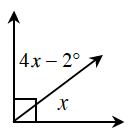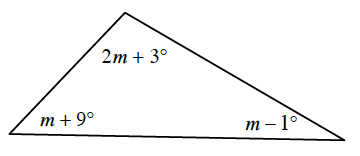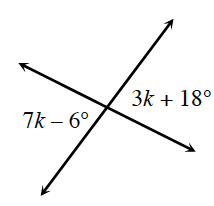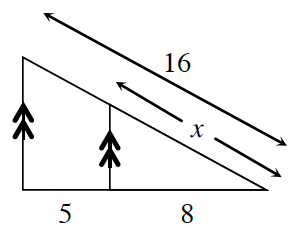### Home > INT2 > Chapter 7 > Lesson 7.1.3 > Problem7-45

7-45.

Examine the geometric relationships in each of the diagrams below. For each one, write an equation and solve for the value of the variable. Name all geometric relationships or theorems that you use.

1.What is the sum of the measure of these angles?

$x = 18.4^\circ$ (complementary angles)

1.What is the sum of the measure of the angles of a triangle?

1.What is true about the measures of vertical angles?

1.These are similar triangles, so write a proportion using the ratios of the corresponding sides.

$\frac{x}{16}=\frac{8}{13}$